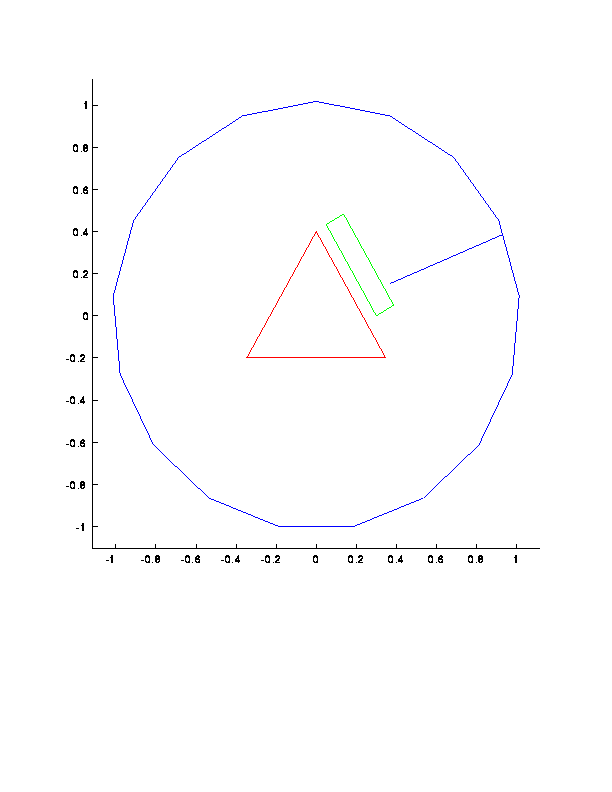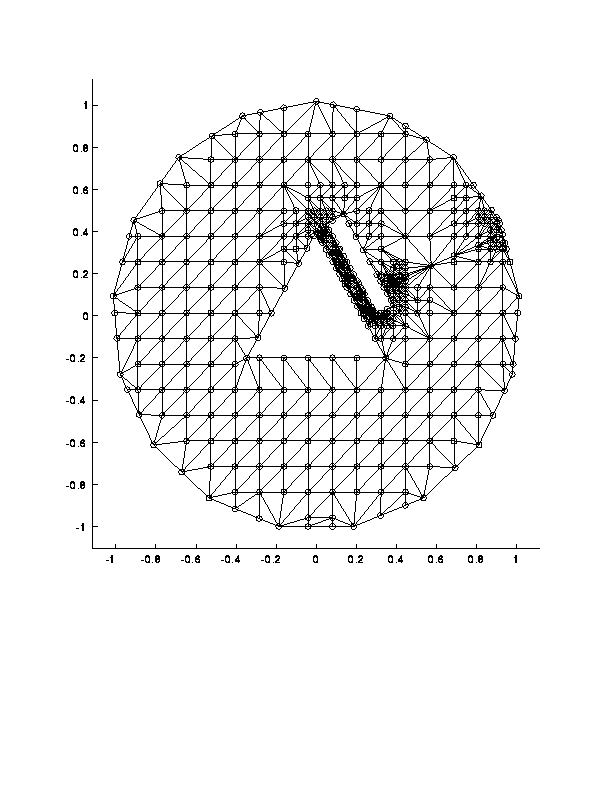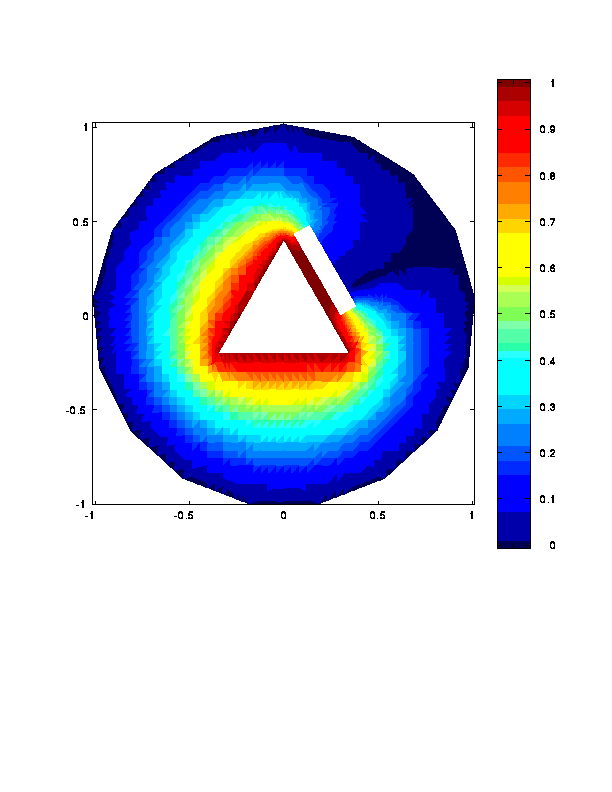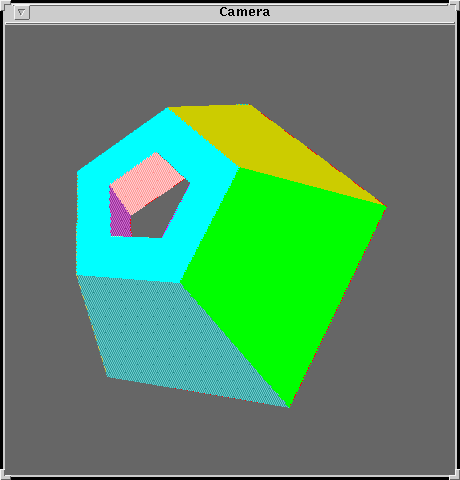# QMG overview

Consider the following problem: you have some kind of mechanical part. One facet of the part is held at 100 degrees C, an opposite facet is 0 degrees C. All other facets are thermally insulated. What is the equilibrium temperature distribution on the interior of the part? Where are the largest temperature gradients? This problem is called a boundary value problem. One popular way to solve boundary value problems is the finite element method.

From the point of view of software development, the three most difficult aspects of finite element analysis are the geometric modeling tools, the mesh generator, and the finite element linear system solver. The QMG package is intended to address geometric modeling and mesh generation in three dimensions. It also has a somewhat elementary finite element solver; the sparse matrix solution is handled by Matlab's backslash operator.

The QMG package is embedded in Matlab. The user interacts with it by typing ordinary Matlab commands. All the Matlab functions associated with QMG in matlab begin with the prefix ``gm,'' which is short for ``geometry.'' The functions that begin with ``gm_'' are lower-level ``internal'' functions.

Since Matlab supports only one data type (matrices), geometric objects are represented by arrays whose elements are gibberish to Matlab. These arrays are actually encoded C++ objects.

The geometric modeler and mesh generator can handle polyhedral objects only. A polyhedral object is one that can be constructed by a finite sequence of unions and intersections of halfspaces. (A non-polyhedral object can be approximated by a polyhedral object with many small facets. But there is a very significant penalty in terms of performance of the modeler and mesh generator for many small facets.)

Because the QMG package can handle only flat objects, and because the performance of the mesh generator is not as good as what we'd like, the QMG package is not quite ``professional grade'' software. (In addition, unlike a professional software product, the QMG package comes without warranties and without any promise of support to its users.)

Nonetheless, we believe that QMG package will be useful to researchers in computational geometry and numerical analysis. We believe that there is substantial opportunity in experimenting with and improving many of the underlying algorithms, and indeed, we are interested in future research on improvements.

If you are familiar with mesh generation, here are some of the distinguishing features of the QMG mesh generator that may be different from mesh generators you have used previously. On the positive side,

• The QMG mesh generator is fully automatic, meaning that no human intervention is necessary to guide the mesh generation or preprocess the domain once you have constructed it with the modeler.
• The QMG mesh generator handles complicated topology. The domain can have holes or internal boundaries, and it can be disconnected. It is OK to have more than three facets meet at a single vertex.
• The QMG mesh generator has certain theoretical guarantees.
On the negative side,
• As mentioned above, the QMG mesh generator can triangulate polyhedral objects only.
• The QMG mesh generator generates an ``unstructured'' mesh, meaning that the mesh is composed of triangles (in 2D) or tetrahedra (in 3D) with no regular pattern. Structured meshes are preferable for some applications: these are meshes with a global cartesian product structure on the mesh nodes. Structured meshes are easier for computation and are preferable for problems with a strong anisotropy (such as a boundary layer in a high-speed flow.) But they are much more difficult to fit into complicated geometries. Unstructured meshes are typically used for isotropic problems that don't have boundary layers.

Structured meshes are a focus of the National Grid Project at the Engineering Research Center at Mississippi State University.

• The QMG mesh generator is not compatible with any existing commercial CAD file format (but this may change).

A user of the QMG package typically has two tasks to accomplish:

• Geometric modeling
• Finite element analysis

The geometric model is typically constructed in QMG by calling some of the basic constructors, applying various transformations to the constructed objects, and then applying boolean operations (set union, set difference, etc) to the objects, yielding the final desired shape.

For three-dimensional geometric modeling, graphics are crucial. We rely on the Geomview package from the Geometry Center, University of Minnesota for rendering. If you have your own program that renders polygons in 3D, you can connect it with your own software to QMG.

For the finite element analysis, the user must call the mesh generator, set up the desired boundary conditions, and call the solver program. The boundary conditions are arbitrary matlab functions. They are associated with faces of the domain as property-value pairs of the faces.

## A 2-dimensional example

Here is an example of solving a two-dimensional boundary value problem using QMG. This is test file `test1` in the examples directory. The domain is a regular polygon with two holes and one slit (an internal boundary). The boundary conditions are u=1 on the exterior face and the slit, u=0 on the triangular hole, du/dn=0 (insulated) on the rectangular hole. The user's matlab commands are prefixed with >>.

``````

%% This is an example of a 2D finite element problem with
%% an SL internal boundary.

>> [s,w] = unix('uname -a');
disp(w)
SunOS syn 4.1.3_U1 1 sun4m

>> [s,w] = unix('hostname');
>> disp(w)
syn

>> clock1
1995 may 2   18:24:20

%% Construct an approximately circular region with a triangular hole,
%% a rectangular hole, and a slit.

>> p1 = gmpolygon(17);

>> if exist('interactive')
>>   pause
>> end

%% Rotate the slit so that it is oblique and also subtract
%% it to form an internal boundary.

>> [p,seg,square,c] = gmbasics;
>> eseg = gmembed(seg);
>> t1 = gmrotate(pi/8);
>> t2 = gmtranslate([.4,0]);
>> eseg1 = gmapply(gmcompose(t1,t2), eseg);
>> p2 = gmsubtract(p1, eseg1);
>> if exist('interactive')
>>   pause
>> end

%% Shrink the triangle and make the triangular hole

>> tri = gmpolygon(3);
>> tri1 = gmapply(gmdilate(.2,.2), tri);
>> p3 = gmsubtract(p2, tri1);

>> if exist('interactive')
>>   pause
>> end

%% Make a rectangular hole

>> t1 = gmtranslate(.3,0);
>> t2 = gmrotate(pi/6);
>> t3 = gmdilate(.1,.5);
>> rect1 = gmapply(gmcompose(t1,gmcompose(t2,t3)), square);
>> domain = gmsubtract(p3, rect1);

%% See what we have.

>> if exist('interactive')
>>   domaincolor = gmrndcolor(domain);
>>   gmviz(domaincolor)
>>   gmshowcolor(domaincolor)
>>   pause
>> end

%% Assign boundary conditions.  Put dir. condition of 1
%% on the triangular hole.  Put dir
%% condition of 0 on the outer boundary and the slit.

>> for i = 0:17
>>   domain = gm_addpropval(domain,1,i,'bc', '<d gm_zero>');
>> end

>> for i = 18:20
>>   domain = gm_addpropval(domain,1,i,'bc', '<d gm_one>');
>> end

%%  Put Neumann cond 0 on the rectangular slit.

>> for i = 21:24
>>   domain = gm_addpropval(domain,1,i,'bc', '<n gm_zero>');
>> end

%% Generate a mesh.  Specify 0.2 as the refinement level.

>> clock1
1995 may 2   18:24:26
>> mesh = gmmeshgen(domain,'point2');
>> clock1
1995 may 2   18:24:54

%% Display the properties of the mesh

>> gmget(mesh)
Type =              simpcomplex
EmbeddedDim =       2
IntrinsicDim =      2
NumSimplices =      856
NumVertices =       487
Vertices =          [ (487 by 2) ]
Simplices =         [ (856 by 3) ]
SourceSpec =        [ (487 by 2) ]
>> gmchecktri(domain,mesh);

Maximum aspect ratio =      1.427516e+01 achieved in triangle 554, vertices =
62 ( 0.574109 0.237804)  source = 1/17
67 ( 0.445326 0.184460)  source = 1/17
376 ( 0.445326 0.195029)  source = 2/0
Maximum global side length =       2.236968e-01
Minimum global altitude =       2.665202e-03

%% Display the mesh
>> if exist('interactive')
>>   gmviz(mesh,'w')
>>   pause
>> end

%% Solve the boundary value problem.  Conductivity=1 everywhere assumed.

>> clock1
1995 may 2   18:24:56
>> u = gmfem(domain, mesh);
Conductivity on toplev face 0 = gm_one
facet 0 btype = /d/ bfunc = /gm_zero/
facet 1 btype = /d/ bfunc = /gm_zero/
facet 2 btype = /d/ bfunc = /gm_zero/
facet 3 btype = /d/ bfunc = /gm_zero/
facet 4 btype = /d/ bfunc = /gm_zero/
facet 5 btype = /d/ bfunc = /gm_zero/
facet 6 btype = /d/ bfunc = /gm_zero/
facet 7 btype = /d/ bfunc = /gm_zero/
facet 8 btype = /d/ bfunc = /gm_zero/
facet 9 btype = /d/ bfunc = /gm_zero/
facet 10 btype = /d/ bfunc = /gm_zero/
facet 11 btype = /d/ bfunc = /gm_zero/
facet 12 btype = /d/ bfunc = /gm_zero/
facet 13 btype = /d/ bfunc = /gm_zero/
facet 14 btype = /d/ bfunc = /gm_zero/
facet 15 btype = /d/ bfunc = /gm_zero/
facet 16 btype = /d/ bfunc = /gm_zero/
facet 17 btype = /d/ bfunc = /gm_zero/
facet 18 btype = /d/ bfunc = /gm_one/
facet 19 btype = /d/ bfunc = /gm_one/
facet 20 btype = /d/ bfunc = /gm_one/
facet 21 btype = /n/ bfunc = /gm_zero/
facet 22 btype = /n/ bfunc = /gm_zero/
facet 23 btype = /n/ bfunc = /gm_zero/
facet 24 btype = /n/ bfunc = /gm_zero/
>> clock1
1995 may 2   18:24:60

%% Plot the FEM solution

>> if exist('interactive')
>>   figure
>>   colormap(jet)
>>   gmplot(mesh,u)
>>   colorbar
>> end
>> diary off

```
```
We now illustrate what this program produced graphically. The original brep (``brep'' is the term used for geometric objects in QMG) looks like this. The edges have been colored so that green corresponds to the insulated faces, red to the u=1 boundary condition, and blue to the u=0 boundary condition.The mesh produced by the mesh generator looks like this:As seen from the above trace, the worst aspect ratio occurring in this mesh is about 14. It is obvious from looking at this mesh that the mesh generator could ``do better'' in the sense that there are nodes that could be locally displaced to improve the overall quality of the mesh.

We have not implemented any heuristic improvement techniques because our focus has been on three dimensions. In three dimensions we do not know how to heuristically improve meshes.

Finally, the color plot of the finite element solution with mixed boundary conditions looks like this. (Note: in Matlab and postscript the color map has more gradations than the following gif image.)## A 3-dimensional example

Here is an example of using the modeler to construct a reasonably interesting geometric object. This is test5 in the test set. Then a mesh generated. No BVP is solved in this test case (but other tests in the set involve 3D finite element solution). You can see from the trace what the running time for this example is, and what the properties of the resulting mesh were.
``````

%% In this test, we'll construct a mesh for an interesting
%% 3D shape, that is a tube with an annular pentagonal cross-section.
%% Then we'll triangulate it.  We won't try a boundary value problem
%% this time.

>> [s,w] = unix('uname -a');
>> disp(w)
SunOS syn 4.1.3_U1 1 sun4m

>> [s,w] = unix('hostname');
>> disp(w)
syn

>> clock1
1995 apr 12   16:58:26

>> base = gmpolygon(5);
>> pyr = gmcone(base,[0,0,2]);
>> pyr1 = gmapply(gmtranslate(0,0,-.5),pyr);
>> pyr2 = gmsubtract(pyr,pyr1);
>> pyr3 = gmapply(gmtranslate(0,0,1),pyr);
>> tubeobj = gmsubtract(pyr2,pyr3);
>> gmget(tubeobj)
Type =              brep
EmbeddedDim =       3
IntrinsicDim =      3
Level0Size =        20
Level1Size =        30
Level2Size =        12
Level3Size =        1
>> tubeobj = gmrndcolor(tubeobj);
>> if exist('interactive') & exist('password')==2 & exist('gm_tclexecute') == 3
>>   eval('gmgeomview(tubeobj);');
>>   pause
>> end

%% Generate a coarse mesh for this object

>> clock1
1995 apr 12   16:58:33
>> mesh = gmmeshgen(tubeobj, 'point4');
clock1
>> 1995 apr 12   17:27:10

>> gmget(mesh)
Type =              simpcomplex
EmbeddedDim =       3
IntrinsicDim =      3
NumSimplices =      20167
NumVertices =       4599
Vertices =          [ (4599 by 3) ]
Simplices =         [ (20167 by 4) ]
SourceSpec =        [ (4599 by 2) ]
>> gmchecktri(tubeobj,mesh);

Maximum aspect ratio =      1.122575e+03 achieved in triangle 10482, vertices =
132 ( 0.622289 -0.511844 0.000000)  source = 1/11
1464 ( 0.625767 -0.367011 0.082894)  source = 2/7
3343 ( 0.625767 -0.367011 0.086797)  source = 3/0
4247 ( 0.625767 -0.444781 0.086797)  source = 3/0
Maximum global side length =       4.268270e-01
Minimum global altitude =       1.504445e-04

>> diary off

```
```
The image of the object constructed as shown by Geomview is# Acknowledgements

I received very good advice on C++ programming from my colleague Bard Bloom. Unfortunately, I didn't follow Bard's advice on good C++ program practices, so the ugliness of the source code is my fault. My colleague Paul Chew gave me advice on computational geometry. My colleague Brian Smith introduced me to TCL. And of course, the mesh generator would not exist without the collaborative research effort with Scott Mitchell to develop the underlying algorithm.

This work was supported by the Division of Advanced Scientific Computing of the National Science Foundation through a Presidential Young Investigator grant. Matching funds were received from Xerox, AT&T, Tektronix, IBM, and Sun Microsystems (not all during the same year).

This documentation is written by Stephen A. Vavasis and is copyright (c) 1995 by Cornell University. Permission to reproduce this documentation is granted provided this notice remains attached. There is no warranty of any kind on this software or its documentation. See the accompanying file 'Copyright' for a full statement of the copyright.

example.html,v 1.1.1.1 1995/05/05 01:15:55 vavasis Exp

Stephen A. Vavasis, Computer Science Department, Cornell University, Ithaca, NY 14853, vavasis@cs.cornell.edu